## Method

The Fastest Visual Ego-motion Algorithm in the West [CUDA-EgoMotion]

Submitted on 28 Aug. 2018 22:23 by
Abiel Aguilar-González (National Institute of Astrophysics, Optics and Electronics (INAOE), Mexico)

 Running time: .001 s Environment: GPU @ 2.5 Ghz (Matlab)

 Method Description: Ego-motion based in look-up tables Parameters: search size = 10 patch size = 10 Latex Bibtex: @article{aguilar2019fastest, title={The Fastest Visual Ego-motion Algorithm in the West}, author={Aguilar-González, Abiel and Arias- Estrada, Miguel and Berry, Fran{\c{c}}ois and J. A.de Jesús Osuna-Coutiño}, journal={Microprocessors and Microsystems}, year={2019}, publisher={Elsevier} }

## Detailed Results

From all test sequences (sequences 11-21), our benchmark computes translational and rotational errors for all possible subsequences of length (5,10,50,100,150,...,400) meters. Our evaluation ranks methods according to the average of those values, where errors are measured in percent (for translation) and in degrees per meter (for rotation). Details for different trajectory lengths and driving speeds can be found in the plots underneath. Furthermore, the first 5 test trajectories and error plots are shown below.

## Test Set Average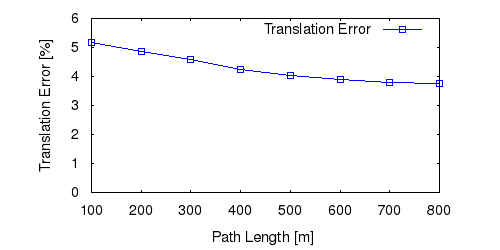This figure as: png eps pdf txt gnuplot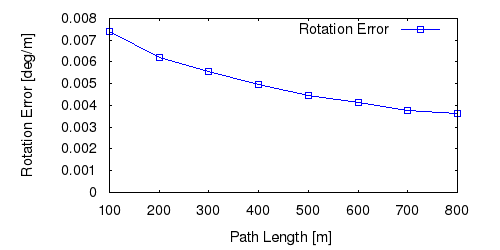This figure as: png eps pdf txt gnuplot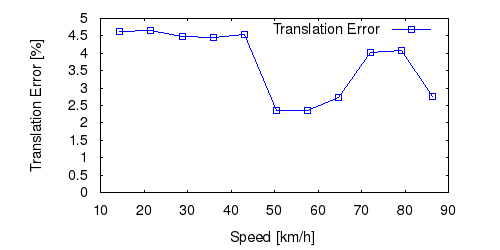This figure as: png eps pdf txt gnuplot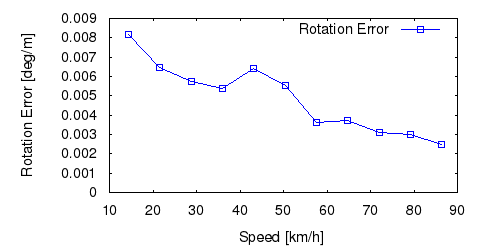This figure as: png eps pdf txt gnuplot

## Sequence 11This figure as: png eps pdf txt gnuplot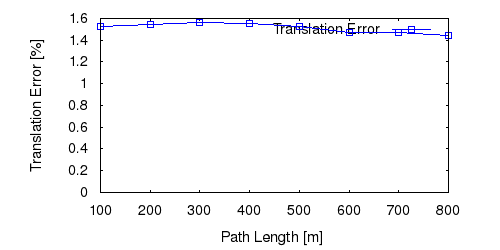This figure as: png eps pdf txt gnuplot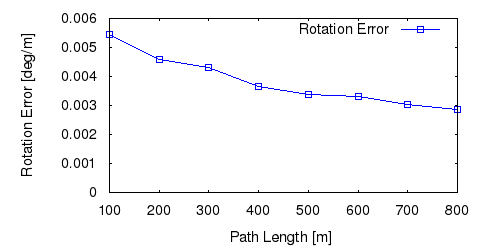This figure as: png eps pdf txt gnuplot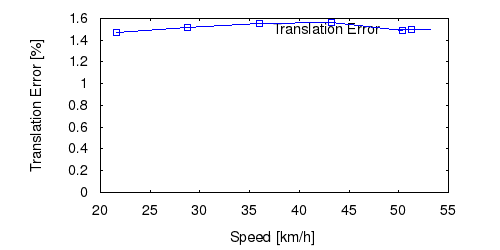This figure as: png eps pdf txt gnuplot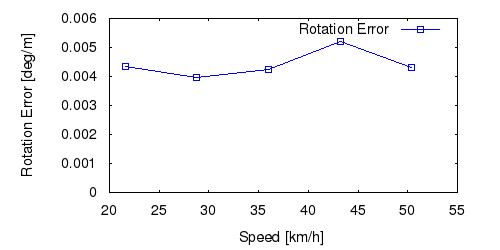This figure as: png eps pdf txt gnuplot

## Sequence 12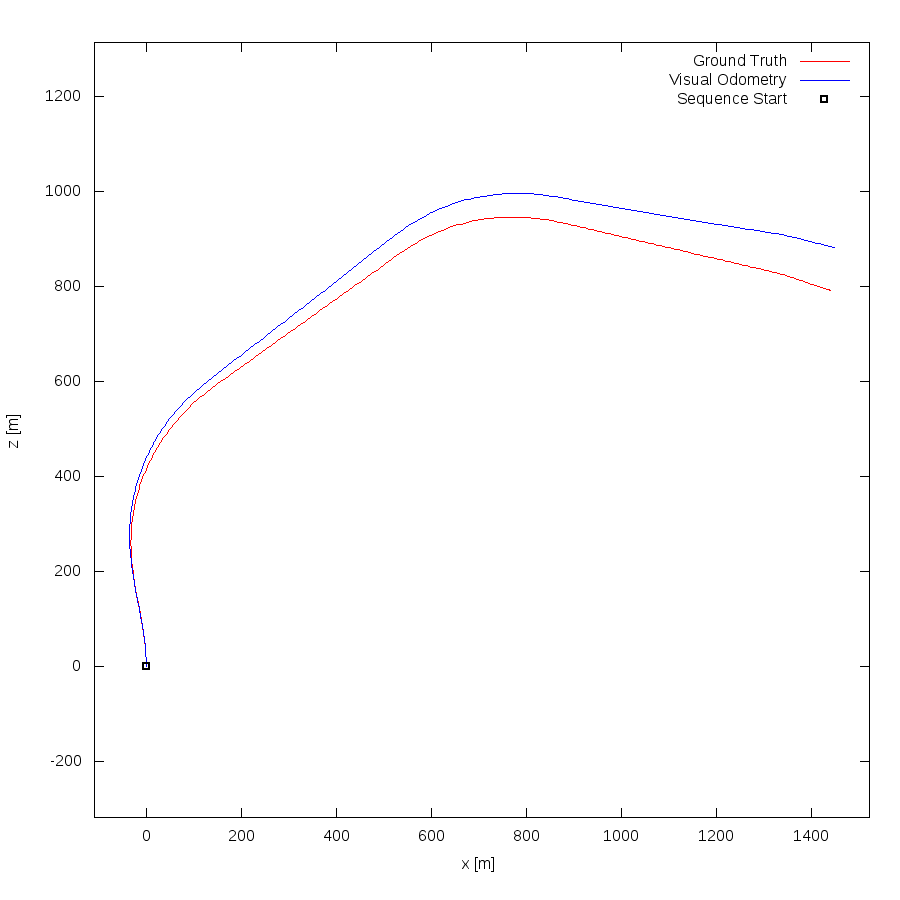This figure as: png eps pdf txt gnuplotThis figure as: png eps pdf txt gnuplot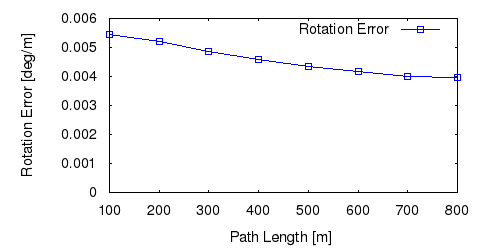This figure as: png eps pdf txt gnuplot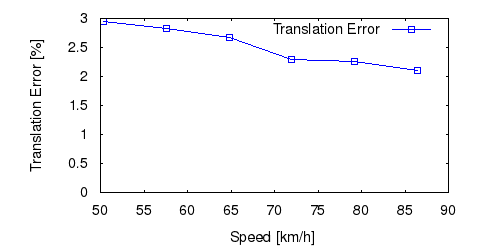This figure as: png eps pdf txt gnuplotThis figure as: png eps pdf txt gnuplot

## Sequence 13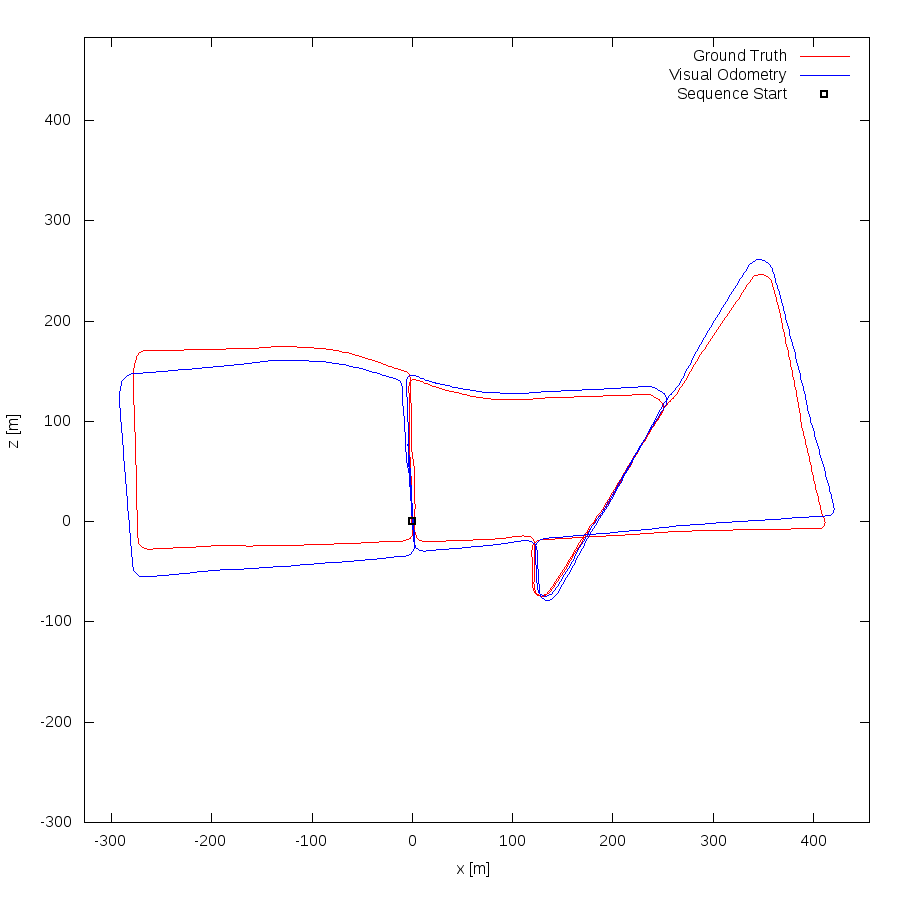This figure as: png eps pdf txt gnuplot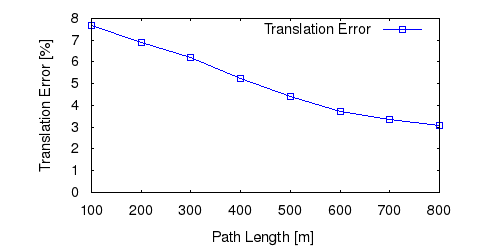This figure as: png eps pdf txt gnuplot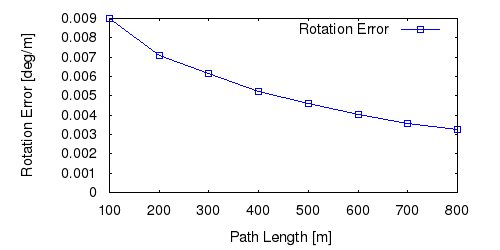This figure as: png eps pdf txt gnuplotThis figure as: png eps pdf txt gnuplotThis figure as: png eps pdf txt gnuplot

## Sequence 14This figure as: png eps pdf txt gnuplot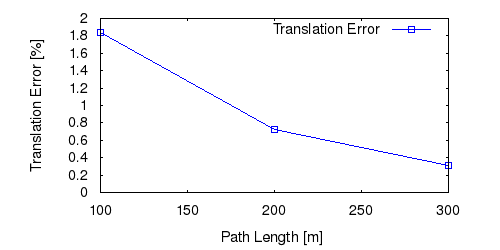This figure as: png eps pdf txt gnuplotThis figure as: png eps pdf txt gnuplot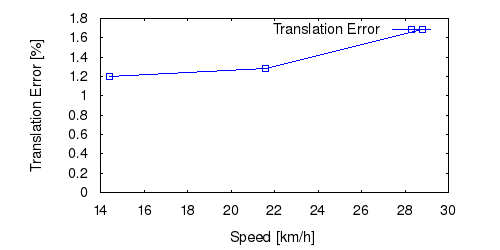This figure as: png eps pdf txt gnuplot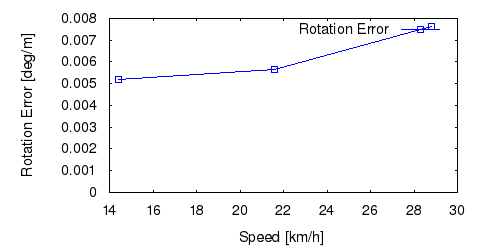This figure as: png eps pdf txt gnuplot

## Sequence 15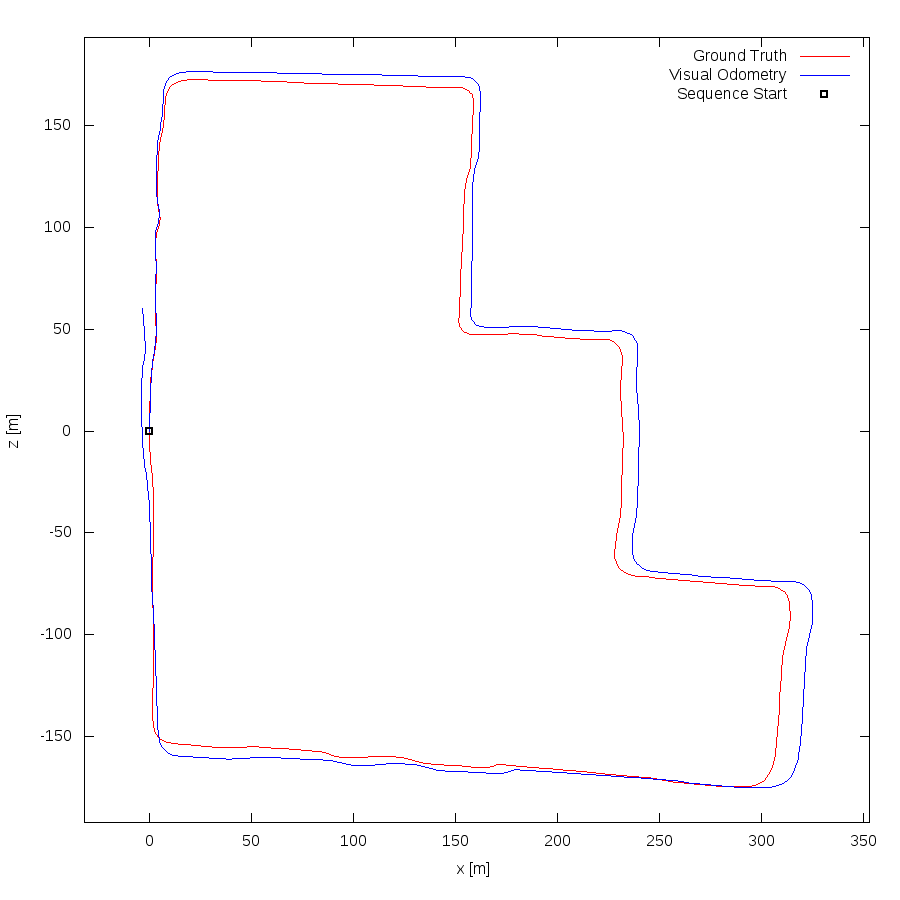This figure as: png eps pdf txt gnuplot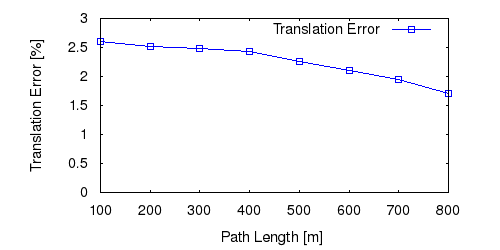This figure as: png eps pdf txt gnuplotThis figure as: png eps pdf txt gnuplot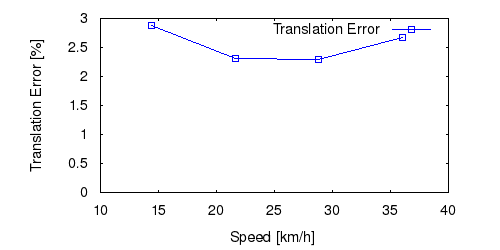This figure as: png eps pdf txt gnuplotThis figure as: png eps pdf txt gnuplot